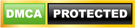Blog

# Data Types in PHP

• ###### | 2908 Ratings

DATA TYPES

PHP supports mainly 8 kinds of data types among which four are stable data types, two are compound data types and another two are special data types

STABLE DATA TYPES:

i) int (or) integer       ii) Float (or) real (or) decimal         iii) Boolean data types

iv) string data types

i) int (or) integer:- all non decimal values . range in between 2(32-1) to -2(32-1)Checkout PHP Interview Questions

ii) Float (or) real (or) decimal: –all decimal values. Range in between 10(38) to 10(-38)iii) Boolean data types:-Boolean data types written in the form of true (or) falseiv) String data types: – a collection of characters enclose in between single (or) double cotations  are known as string data typesCOMPOUND DATA TYPES:-

compound data types again two types

1. Array data type
2. Object data type

Related Page: PHP Interview Questions

1. ARRAY DATA TYPE:

Array data type which can hold more than one value. In php we have mainly 3 kinds of arrays

i) Numerical array
ii) Associative array
iii) Mixed array
Declaring an array in php can be done by two types

• array as the function
• array as a square bracket

i) Numerical array: –

A numeric array stores each array element with a numeric index.
There are two methods to create a numeric array.

1. In the following example the index are automatically assigned (the index starts at 0):

\$cars=array(“Saab”,”Volvo”,”BMW”,”Toyota”);

2. In the following example we assign the index manually:ii) Associative array: – An associative array, each ID key is associated with a value.

When storing data about specific named values, a numerical array is not always the best way to do it.
With associative arrays we can use the values as keys and assign values to them.

Example 1

In this example we use an array to assign ages to the different persons:

\$ages = array(“Peter”=>32, “Quagmire”=>30, “Joe”=>34);

Example 2

This example is the same as example 1, but shows a different way of creating the array:The code above will output:

Peter is 32 years old.

iii) Mixed array: – mixed array is nothing but combination of numerical & associative array  are known as mixed array

Ex:- mixedarrays.php2. OBJECT DATATYPE:

object is an instance of class. Class can be denoted with in class name. Collection of variables and functions are known as class. Calling class name should be create an object variable then that particular variable is known as object variableSPECIAL DATA TYPES:

Special data types are again two types

i) Resource data types
ii) Null data types

Related Page: Cookies, Sessions And Buffer In PHP

i) Resource data types:- resource data type is calling an external variable value into another variable using (&) resource variable
ii) Null data types:- this is the pre-defined key-word data type. When a variable not initialize and by asking through printing method. This will result in null value

ex: –